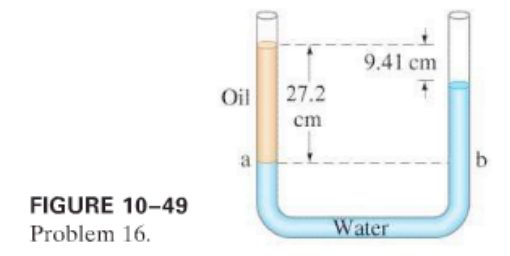×
Get Full Access to Physics: Principles With Applications - 6 Edition - Chapter 10 - Problem 16p
Get Full Access to Physics: Principles With Applications - 6 Edition - Chapter 10 - Problem 16p

×

Water and then oil (which don’t mix) are poured into aISBN: 9780130606204 3

Solution for problem 16P Chapter 10

Physics: Principles with Applications | 6th Edition

• Textbook Solutions
• 2901 Step-by-step solutions solved by professors and subject experts
• Get 24/7 help from StudySoup virtual teaching assistantsPhysics: Principles with Applications | 6th Edition

4 5 1 379 Reviews
27
0
Problem 16P

Water and then oil (which don’t mix) are poured into a U-shaped tube, open at both ends. They come to equilibrium as shown in Fig. 10–49. What is the density of the oil? [Hint: Pressures at points a and b are equal. Why?]Step-by-Step Solution:
Step 1 of 3

ANSWER:STEP 1:-The speed of the fastest human is 11 m/s.Let’s say, his mass is “m”.The kinetic energy will be, K.E = 1/2 m V = 1/2 × m × 11 = 1/2 × m × 121 = 60.5 m jules.The potential energy or the gravitational potential energy he would have at a height “h”, P.E = mgh--------------(1)According to our requirement, both the energies should be the same. So, the height at whichthe condition would satisfy is, K.E = P.E 60.5 m = mgh = m × 9.8 × h h = 60.5 m / 9.8 m h = 6.172 meters.CONCLUSION:So, at a height of 6.172 meters the sprinter will attain the potential energy equal to the kineticenergy of world’s fastest man running at the speed of 11 m/s.

Step 2 of 3

Step 3 of 3

ISBN: 9780130606204

The answer to “?Water and then oil (which don’t mix) are poured into a U-shaped tube, open at both ends. They come to equilibrium as shown in Fig. 10–49. What is the density of the oil? [Hint: Pressures at points a and b are equal. Why?]” is broken down into a number of easy to follow steps, and 43 words. Physics: Principles with Applications was written by and is associated to the ISBN: 9780130606204. Since the solution to 16P from 10 chapter was answered, more than 568 students have viewed the full step-by-step answer. This full solution covers the following key subjects: oil, mix, Density, don, ends. This expansive textbook survival guide covers 35 chapters, and 3914 solutions. The full step-by-step solution to problem: 16P from chapter: 10 was answered by , our top Physics solution expert on 03/03/17, 03:53PM. This textbook survival guide was created for the textbook: Physics: Principles with Applications, edition: 6.

Unlock Textbook Solution